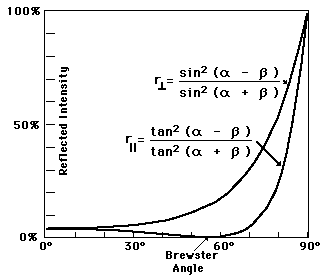# Brewster angle & polarised light

## Homework Statement

A beam of polarised light is incident at Brester angle to a piece of glass. Which is true?

The ans is the refracted ray is polarized in the plane of incident beam , and the reflected ray is polarised in the plane normal to the plane of incident beam. Or
The reflected ray is polarized in the plane of incident beam , and the refracted ray is polarised in the plane normal to the plane of incident beam?
For me the above statement is correct… I need someone to verify my ans….

## The Attempt at a Solution

ehild
Homework Helper
Which answer above is true? Is any of them true?
How is the Brewster angle defined?

•desmond iking
brewster angle is defined as when the the incident angle of light is equal to polarising angle (brewster) , the reflected lught will be completely plane polarised.

my ans is The ans is the refracted ray is polarized in the plane of incident beam , and the reflected ray is polarised in the plane normal to the plane of incident beam.

is it correct?

ehild
Homework Helper
brewster angle is defined as when the the incident angle of light is equal to polarising angle (brewster) , the reflected lught will be completely plane polarised.

my ans is The ans is the refracted ray is polarized in the plane of incident beam , and the reflected ray is polarised in the plane normal to the plane of incident beam.

is it correct?

No, it is wrong. See picture. It show the reflectance for both the parallel and perpendicular polarized light in terms of the angle of incidence. . It is zero at the Brewster angle for which polarization? So how can be the reflected light polarized at the Brewster angle?
The incident energy is partly reflected, partly transmitted (refracted) . What can you say about the polarization of the refracted light?The reflected ray is polarized in the plane of incident beam , and the refracted ray is polarised in the plane normal to the plane of incident beam...this is the correct ans?

ehild
Homework Helper
The reflected ray is polarized in the plane of incident beam , and the refracted ray is polarised in the plane normal to the plane of incident beam...this is the correct ans?

NO. Look at the figure. r means reflection, what is at the Brewster angle in case of parallel polarization? r with the two small parallel lines means the reflectance at parallel polarization. Parallel and perpendicular are meant with respect to the plane of incidence, defined by the incoming ray and the normal of the interface.